# Texas Go Math Grade 4 Lesson 8.7 Answer Key Multiply 2-Digit Numbers

Refer to our Texas Go Math Grade 4 Answer Key Pdf to score good marks in the exams. Test yourself by practicing the problems from Texas Go Math Grade 4 Lesson 8.7 Answer Key Area Multiply 2-Digit Numbers.

## Texas Go Math Grade 4 Lesson 8.7 Answer Key Multiply 2-Digit Numbers

Essential Question

How can you use the strategy draw a diagram to solve multi-step multiplication problems?
To use this strategy, we draw a box (the number of columns and rows depend on the number of digits in the factors), and then write the expanded forms of the factors.

Unlock the ProblemDuring the 2010 Great Backyard Bird Count, an average of 42 bald eagles were counted in each of 20 locations throughout Alaska. In 2009, an average of 32 bald eagles were counted in each of 26 locations throughout Alaska. Based on this data, how many more bald eagles were counted in 2010 than in 2009?
42 x 20 = 840   in 2010
32 x 26 = 832   in 2009
8 more bald eagles
Explanation:
42 x 20 = 840   in 2010
32 x 26 = 832   in 2009
840 – 832 = 8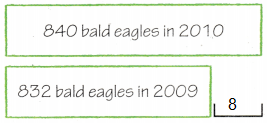What do I need to find?
I need to find ___________ bald eagles were counted in 2010 than in 2009.
How many more bald eagles were counted in 2010 than in 2009?

What information am I given?
In 2010, ___________ locations counted an average of ___________ bald eagles each.
In 2009 ___________ locations counted an average of ___________ bald eagles each.
In 2010, 20 locations counted an average of 42 bald eagles each.
In 2009, 26 locations counted an average of 32 bald eagles each.

Plan

What is my plan or strategy?
I can solve simpler problems.
Find the number of bald eagles counted in ___________ .
Find the number of bald eagles counted in ___________ .
Then draw a strip diagram to compare the ___________ count to the ___________ count.
Find the number of bald eagles counted in 2009 .
Find the number of bald eagles counted in 2010 .
Then draw a strip diagram to compare the 2009 count to the 2010 count.Solve

• First, find the total number of bald eagles counted in 2010.
____________ × ____________
= ____________ bald eagles counted in 2010
42 x 20 = 840 bald eagles counted in   in 2010

• Next, find the total number of bald
eagles counted in 2009.
= ____________ × ____________
= ____________ bald eagles counted in 2009
32 x 26 = 832  bald eagles counted in  in 2009

• Last, draw a strip diagram. I need to subtract.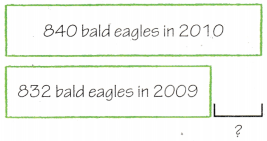840 – 832 = ____________
So, there were ____________ more bald eagles counted in 2010 than in 2009.
42 x 20 = 840   in 2010
32 x 26 = 832   in 2009
840 – 832 = 8
So, there were 8 more bald eagles counted in 2010 than in 2009.Try Another Problem

Prescott Valley, Arizona, reported a total of 29 mourning doves in the Great Backyard Bird Count.
Mesa, Arizona, reported 20 times as many mourning doves as Prescott Valley. If Chandler reported a total of 760 mourning doves, how many more mourning doves were reported in Chandler than in Mesa?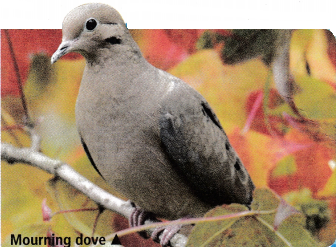What do I need to find?
How many more mourning doves were reported in Chandler than in Mesa

What information am I given?
Prescott Valley, Arizona, reported a total of 29 mourning doves in the Great Backyard Bird Count.
Mesa, Arizona, reported 20 times as many mourning doves as Prescott Valley. If Chandler reported a total of 760 mourning doves,

Plan

What is my plan or strategy?
Using strip drawing to find the answer.
Solve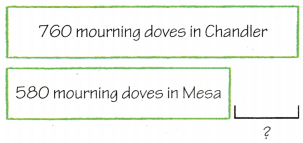Explanation:
29 x 20 = 580
mourning doves in Chandler are 760
mourning doves in Mesa  are  580
finding the difference 760 – 580 = 180

Explanation:
mourning doves in Chandler are 760
mourning doves in Mesa  are  580
finding the difference 760 – 580 = 180

Math Talk

Mathematical Processes
Describe another way you could solve the problem.
Explanation: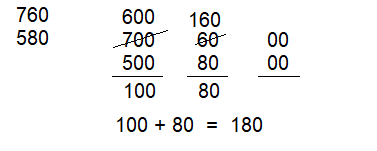Share and Show

Question 1.
Analyze An average of 74 reports with bird counts were turned in each day in June. An average of 89 were turned in each day in July. How many reports were turned in for both months? (Hint: There are 30 days in June and 31 days in July.)
Explanation:
An average of 74 reports with bird counts were turned in each day in June.
In June month there are 30 days.
So, 30 x 74 = 2,220
An average of 89 were turned in each day in July.
In the month of July there are 31 days.
So, 31 x 89 = 2,759
Total reports turned in for both months
2,220 + 2,759 = 4,979

First, write the problem for June.
Explanation:
An average of 74 reports with bird counts were turned in each day in June.
In June month there are 30 days.
So, 30 x 74 = 2,220

Next, write the problem for July.
Explanation:
An average of 89 were turned in each day in July.
In the month of July there are 31 days.
So, 31 x 89 = 2,759

Last, find and add the two products.

____________ reports were turned in for both months.
Explanation:
The information above states,
Total reports turned in June 2,220
Total reports turned in July 2,759
Total reports turned in for both months
2,220 + 2,759 = 4,979

Question 2.
H.O.T. Multi-Step What if an average of 98 reports were turned in each day for the month of June? How many reports were turned in for June?
The month of June has 30 days.
Explanation:
What if an average of 98 reports were turned in each day for the month of June
Number of reports turned in June
98 x 30 = 2,940

Problem Solving

Question 3.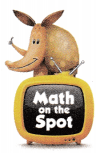H.O.T. Multi-Step On each of Maggie’s bird-watching trips, she has seen at least 24 birds.
If she has taken 4 of these trips each year over the past 16 years, at least how many birds has Maggie seen?
Explanation:
On each of Maggie’s bird-watching trips, she has seen at least 24 birds.
If she has taken 4 of these trips each year over the past 16 years,
16 x 4 = 64
Total birds Maggie has seen past 16 years
64 x 24 = 1,536

Question 4.
H.O.T. Multi-Step Each of 5 bird-watchers reported seeing 15 roseate spoonbills in a day. If they each reported seeing the same number of roseate spoonbills over 14 days, how many would be reported?
1,050 Roseate Spoonbills
Explanation:
Each of 5 bird-watchers reported seeing 15 roseate spoonbills in a day.
15 x 5 = 75
If they each reported seeing the same number of roseate spoonbills over 14 days,
75 x 14 = 1,050

Question 5.
Geoducks and tubeworms are two creatures that live a long time. Pam and Sierra use their ages to describe the ages of these sea creatures. Pam says “I’m 13. Geoducks live to be about 12 times my age.” Sierra says “I’m 11. Tubeworms live to be about 15 times my age.” How many years longer do tubeworms live than geoducks?
(A) 27 years
(B) 10 years
(C) 9 years
(D) 1 year
Option(B)
Explanation:
Pam says “I’m 13.
Geoducks live to be about 12 times my age.
12 x 13 = 156
Sierra says “I’m 11.
Tubeworms live to be about 15 times my age.
15 x 11 = 165
Number of years longer tubeworms live than geoducks
165 – 156 = 10 years

Question 6.
Multi-Step A restaurant buys 32 boxes of 21 packets of mustard each and 26 boxes of 35 packets of ketchup each. How many packets of mustard and ketchup does the restaurant buy?
(A) 304 packets
(B) 238 packets
(C) 1,352 packets
(D) 1,582 packets
Option(D)
Explanation:
A restaurant buys 32 boxes of 21 packets of mustard each
32 x 21 = 672
26 boxes of 35 packets of ketchup each.
26 x 35 = 910
Total packets of mustard and ketchup the restaurant buy
672 + 910 = 1,582

Question 7.
Multi-Step There are 19 cases of 24 cans of vegetable soup each in the school cafeteria. There are 23 cases of 36 cans of chicken soup each in the cafeteria. How many cans of soup are in the school cafeteria?
(A) 321 cans
(B) 1,144 cans
(C) 1,284 cans
(D) 1,274 cans
Option(C)
Explanation:
There are 19 cases of 24 cans of vegetable soup each in the school cafeteria.
19 x 24 = 456
There are 23 cases of 36 cans of chicken soup each in the cafeteria.
23 x 36 = 828
Number of cans of soup are in the school cafeteria
456 + 828 = 1,284

TEXAS Test Prep

Question 8.
Carol is the treasurer of her bird-watching club. The club wants to order shirts for each of the 18 members. If each shirt costs $21, what is the cost for the members’ shirts? (A)$39
(B) $378 (C)$380
(D) $540 Answer: Option(B) Explanation: Carol club wants to order shirts for each of the 18 members. If each shirt costs$21
The total cost for the members’ shirts
18 x 21 = $378 ### Texas Go Math Grade 4 Lesson 8.7 Homework and Practice Answer Key Problem Solving Question 1. One day, Sam’s Sweats sold 83 pairs of sweat pants for$29 each and 75 sweat shirts for $24 each. How much did Sam collect on the two items? Answer: Total amount Sam collect on the two items 6,614 Explanation: Sam’s Sweats sold 83 pairs of sweat pants for$29 each
83 x 2 = 166 sweat pants
Total cost of sweat pants 166 x 29 = 4,814
75 sweat shirts for $24 each. 75 x 24 = 1,800 Total amount Sam collect on the two items 4,814 + 1,800 = 6,614 First write the problem for sweat pants. Answer: One day, Sam’s Sweats sold 83 pairs of sweat pants for$29 each. How much did Sam collect on the Sweat pants?

Next write the problem for sweat shirts.
One day, Sam’s Sweats sold 75 sweat shirts for $24 each. How much did Sam collect on the Sweat shirts? Practice and Homework Lesson 8.7 Answer Key Question 2. Sam’s Sweats sold 68 pairs of sweatpants and 70 sweatshirts the next day. How much did Sam collect on the two items that day? Describe the difference in the sales on the two days. Answer: The difference in the sales on the two days 2,264 Explanation: Sam’s Sweats sold 68 pairs of sweat pants 68 x 2 = 136 Cost of each sweat pant$29
Total cost of sweat pants = 136 x 29 = 3,944
He sold 70 sweat shirts the next day.
Each sweat shirt cost $24 Total cost of sweat shirts = 70 x 24 = 1,680 The difference in the sales on the two days 3,944 – 1,680 = 2,264 Question 3. A factory got an order for 65 cases of shampoo. There are 15 bottles in each case. They have 1,000 bottles of shampoo in stock. Is there enough shampoo in stock to fill the order? If so, how many bottles will be left over? If not, how many more bottles are needed? Answer: Yes, there is enough shampoo in stock to fill the order. 25 bottles are left. Explanation: A factory got an order for 65 cases of shampoo. There are 15 bottles in each case. Total bottles in 65 cases 65 x 15 = 975 They have 1,000 bottles of shampoo in stock. Number of bottles left over 1000 – 975 = Question 4. A store ordered 26 cartons of large dog beds and 22 cartons of small dog beds. There are 12 large dog beds in a carton and 16 small dog beds in a carton. Did the store order more large dog beds or more small dog beds? How many more? Answer: The store order 20 more small dog beds. Explanation: A store ordered 26 cartons of large dog beds, There are 12 large dog beds in a carton. Total large dog beds = 26 x 12 = 312 22 cartons of small dog beds. There are 16 small dog beds in a carton. Total small dog beds = 22 x 16 = 352 The store order 20 more small dog beds. Lesson Check Fill in the bubble completely to show your answer. Question 5. Multi-Step Large postcards come in packages of 20. Small postcards come in packages of 25. A store buys 24 packages of large postcards and 18 packages of small postcards. Which statement is true? (A) The store bought a total of 930 postcards. (B) The store bought 30 more small postcards than large postcards. (C) The store bought a total of 932 postcards. (D) The store bought 68 more large postcards than small postcards. Answer: Option(A) Explanation: Large postcards come in packages of 20. Small postcards come in packages of 25. A store buys 24 packages of large postcards 24 x 20 = 480 18 packages of small postcards. 18 x 25 = 450 Question 6. Multi-Step Sarah can pack 16 books in a box. She has 14 boxes. She has 230 books to pack. Which statement is true? (A) Sarah will have room for 1 more book in the last box she packs. (B) Sarah has exactly the right number of boxes to pack all of the books. (C) Sarah will have 1 box left over after she packs all of the books. (D) Sarah needs 1 more box to pack all of the books. Option(D) Explanation: Sarah can pack 16 books in a box. She has 14 boxes. Total books in 14 boxes = 16 x 14 = 224 She has 230 books to pack. Question 7. Multi-Step Jeans cost$39 a pair. T-shirts cost $14 each. How much does it cost to buy 2 pairs of jeans and 3 T-shirts for each of the 6 members of the Walsh family? (A)$720
(B) $1,908 (C)$870
(D) $626 Answer: Option(A) Explanation: Jeans cost$39 a pair.
T-shirts cost \$14 each.
Each pair of jeans and T-shirt 39 + 14 = 53
The cost of 2 pairs of jeans  39 x 2 = 78 and
3 T-shirts  cost 14 x 3 = 42
Each of the 6 members of the Walsh family
78 + 42 = 120
120 x 6 = 720

Question 8.
Multi-Step There are 472 stuffed bears in stock at a factory. The factory has an order for 35 cartons of bears. There are 16 in a carton. I-low many more stuffed bears do they need to make to fill the order?
(A) 58
(B) 88
(C) 42
(D) 98
Option(B)
Explanation:
There are 472 stuffed bears in stock at the factory.
The factory has an order for 35 cartons of bears.
There are 16 in a carton.
Total bears in carton 35 x 16 = 560
Number of more stuffed bears they need to make to fill the order
560 – 472 = 88

Lesson 8.7 Practice and Homework Answer Key Question 9.
Multi-Step Glenn made 20 trays of 12 muffins each. Laurie made 15 trays of 18 muffins each. How many more muffins did Laurie make?
(A) 40
(B) 20
(C) 10
(D) 30
Option(D)
Explanation:
Glenn made 20 trays of 12 muffins each.
Total muffins  Glenn had 20 x 12 = 240
Laurie made 15 trays of 18 muffins each.
Total muffins Laurie had 15 x 18 = 270
Number of muffins Laurie makes more 270 – 240 = 30

Question 10.
Multi-Step There are 48 crayons in a box. There are 12 boxes in a carton. Mr. Johnson ordered 6 cartons of crayons for the school. How many crayons did he get?
(A) 3,346
(B) 3,446
(C) 3,456
(D) 3,356